##8.1. Consider the the number of pigs slaughtered in Victoria, available in the aus_livestock dataset. ###Use the ETS() function to estimate the equivalent model for simple exponential smoothing. Find the optimal values of α and ℓ0, and generate forecasts for the next four months.

## Series: Count
## Model: ETS(A,N,N)
##   Smoothing parameters:
##     alpha = 0.3221247
##
##   Initial states:
##      l
##  100646.6
##
##   sigma^2:  87480760
##
##      AIC     AICc      BIC
## 13737.10 13737.14 13750.07

This shows that the optimal value for alpha is about 0.32 and the starting value (level estimate for time 0) is 100646.

## # A tibble: 2 x 3
##   .model                                                     term    estimate
##   <chr>                                                      <chr>      <dbl>
## 1 "ETS(Count ~ error(\"A\") + trend(\"N\") + season(\"N\"))" alpha      0.322
## 2 "ETS(Count ~ error(\"A\") + trend(\"N\") + season(\"N\"))" l  100647.
## # A fable: 4 x 4 [1M]
## # Key:     .model 
##   .model                                        Month               Count  .mean
##   <chr>                                         <mth>              <dist>  <dbl>
## 1 "ETS(Count ~ error(\"A\") + trend(\"N\") ~ 2019 Jan  N(95187, 87480760) 95187.
## 2 "ETS(Count ~ error(\"A\") + trend(\"N\") ~ 2019 Feb  N(95187, 96558142) 95187.
## 3 "ETS(Count ~ error(\"A\") + trend(\"N\") ~ 2019 Mar N(95187, 105635524) 95187.
## 4 "ETS(Count ~ error(\"A\") + trend(\"N\") ~ 2019 Apr N(95187, 114712906) 95187.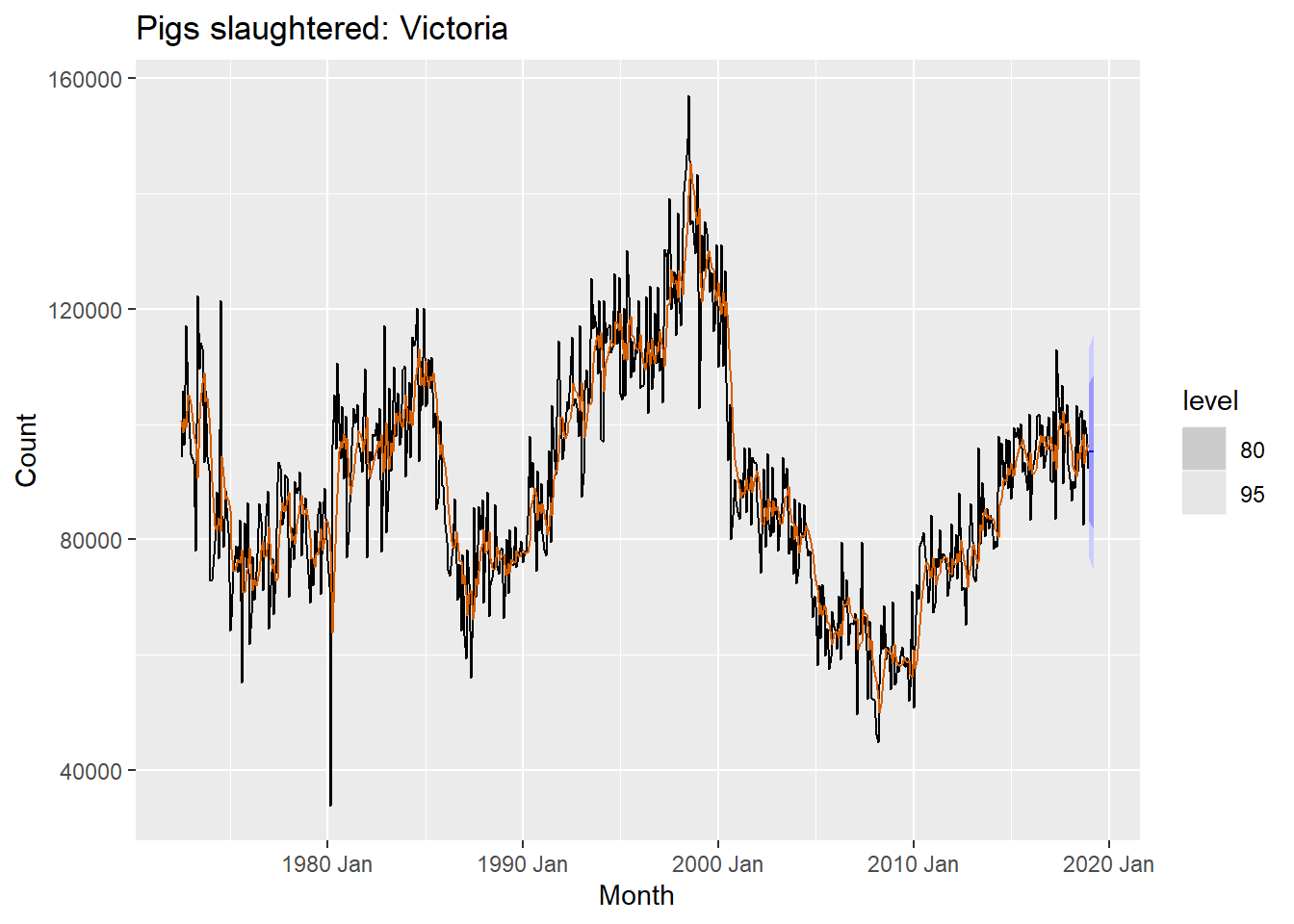Compute a 95% prediction interval for the first forecast using y±1.96s, where s is the standard deviation of the residuals. Compare your interval with the interval produced by R.

## # A tsibble: 1 x 4 [1M]
##      Month  .mean `95%_lower` `95%_upper`
##      <mth>  <dbl>       <dbl>       <dbl>
## 1 2019 Jan 95187.      76855.     113518.

Now we calculate the forecast interval manually as follows:

##  9353.115
##  113518.7
##  76854.45

From the above, we can see that the manually calculated 95% interval for the first forecast period (h=1) matches with the one calculated by the model.

8.5 Data set global_economy contains the annual Exports from many countries. Select one country to analyse.

###a) Plot the Exports series and discuss the main features of the data.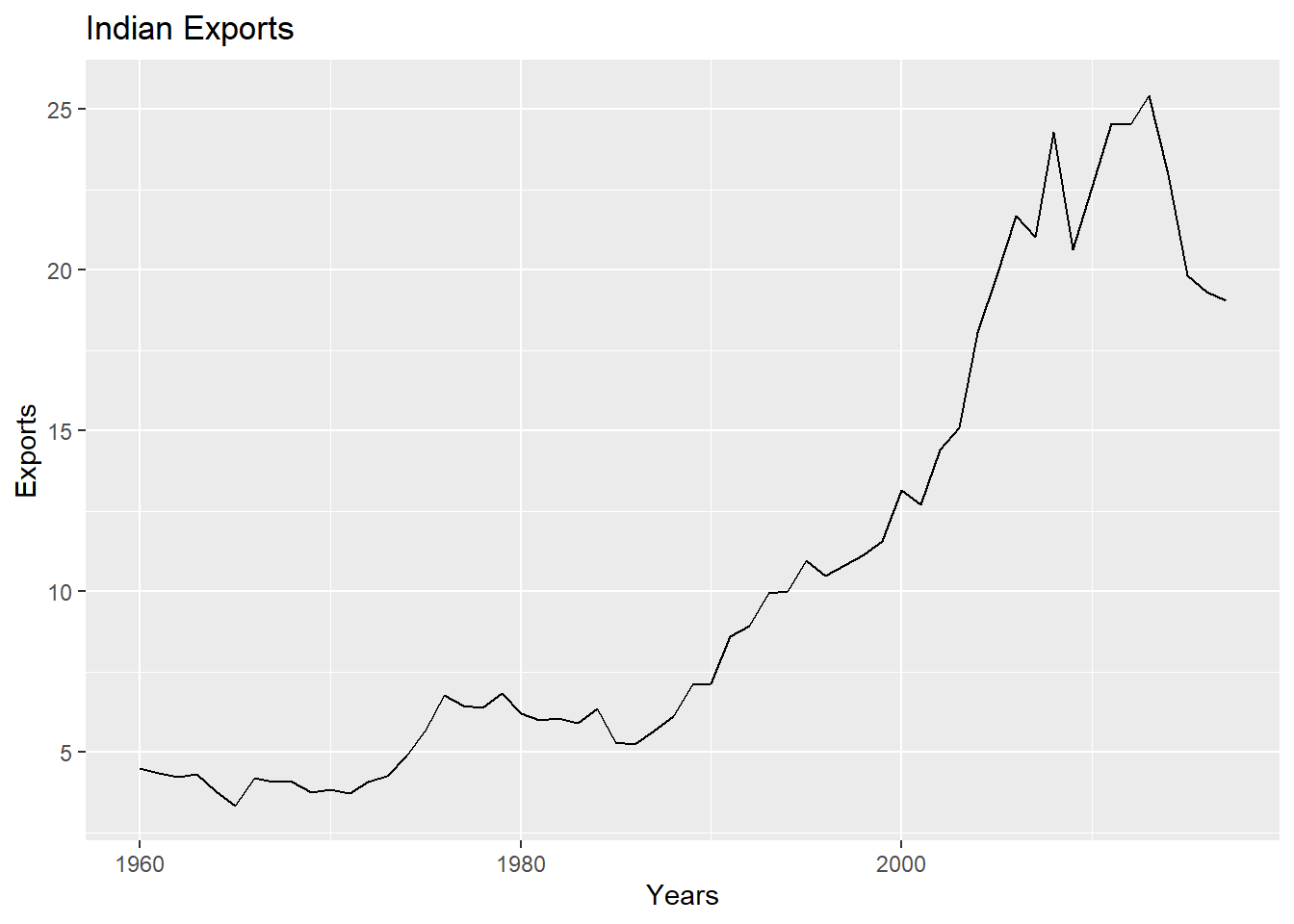The Indian exports time series plot above shows an upward trend for the most part, except for declines during the 2007 to 2009 period and then in 2014 onwards. There is no seasonality.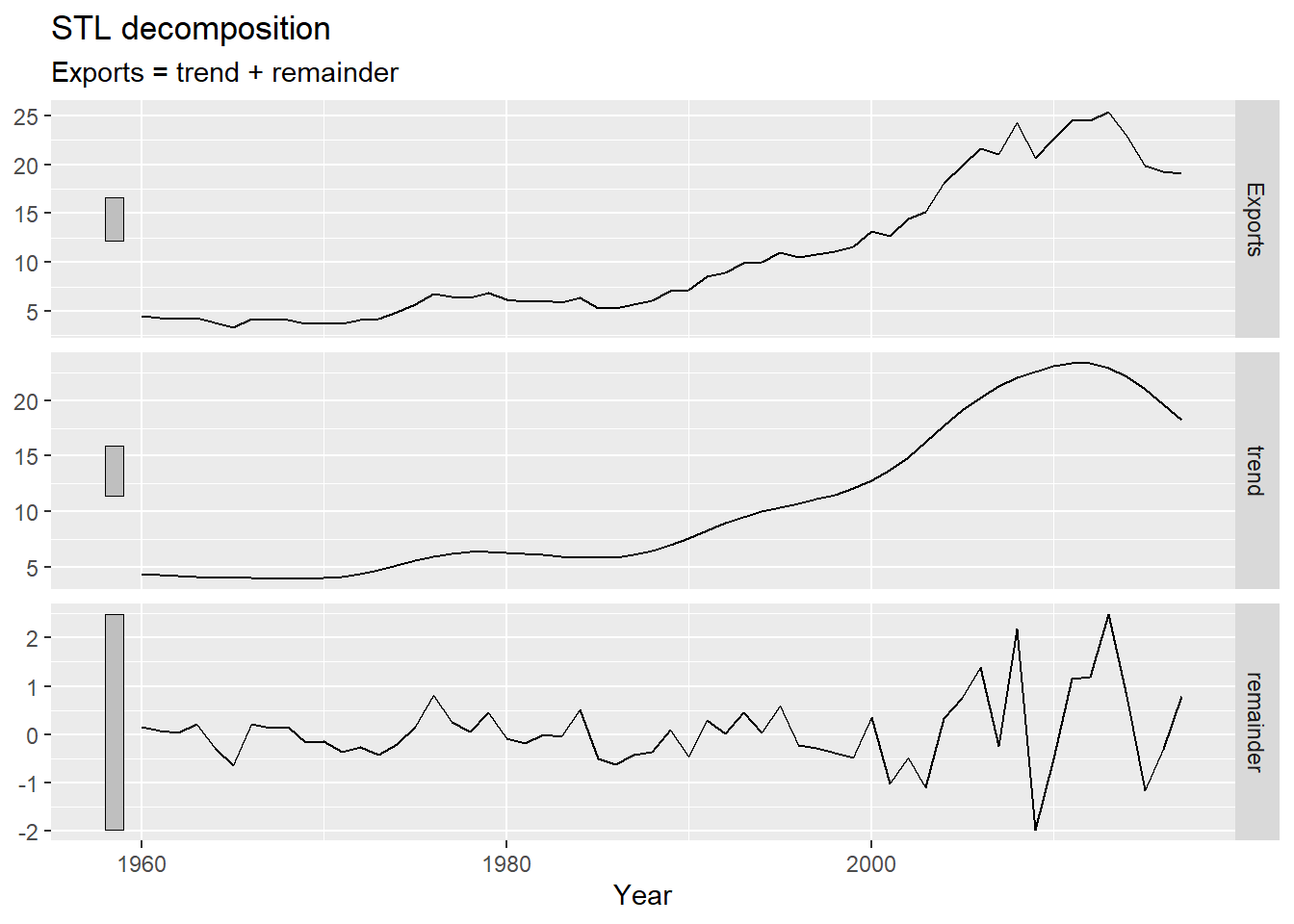The STL decomposition shows a trend component and a remainder, whose variability seems to be increasing over time, and looks like it might be dependent on the level of the time series.

###b) Use an ETS(A,N,N) model to forecast the series, and plot the forecasts.

## Series: Exports
## Model: ETS(A,N,N)
##   Smoothing parameters:
##     alpha = 0.9998965
##
##   Initial states:
##      l
##  4.507901
##
##   sigma^2:  1.4709
##
##      AIC     AICc      BIC
## 261.8526 262.2971 268.0339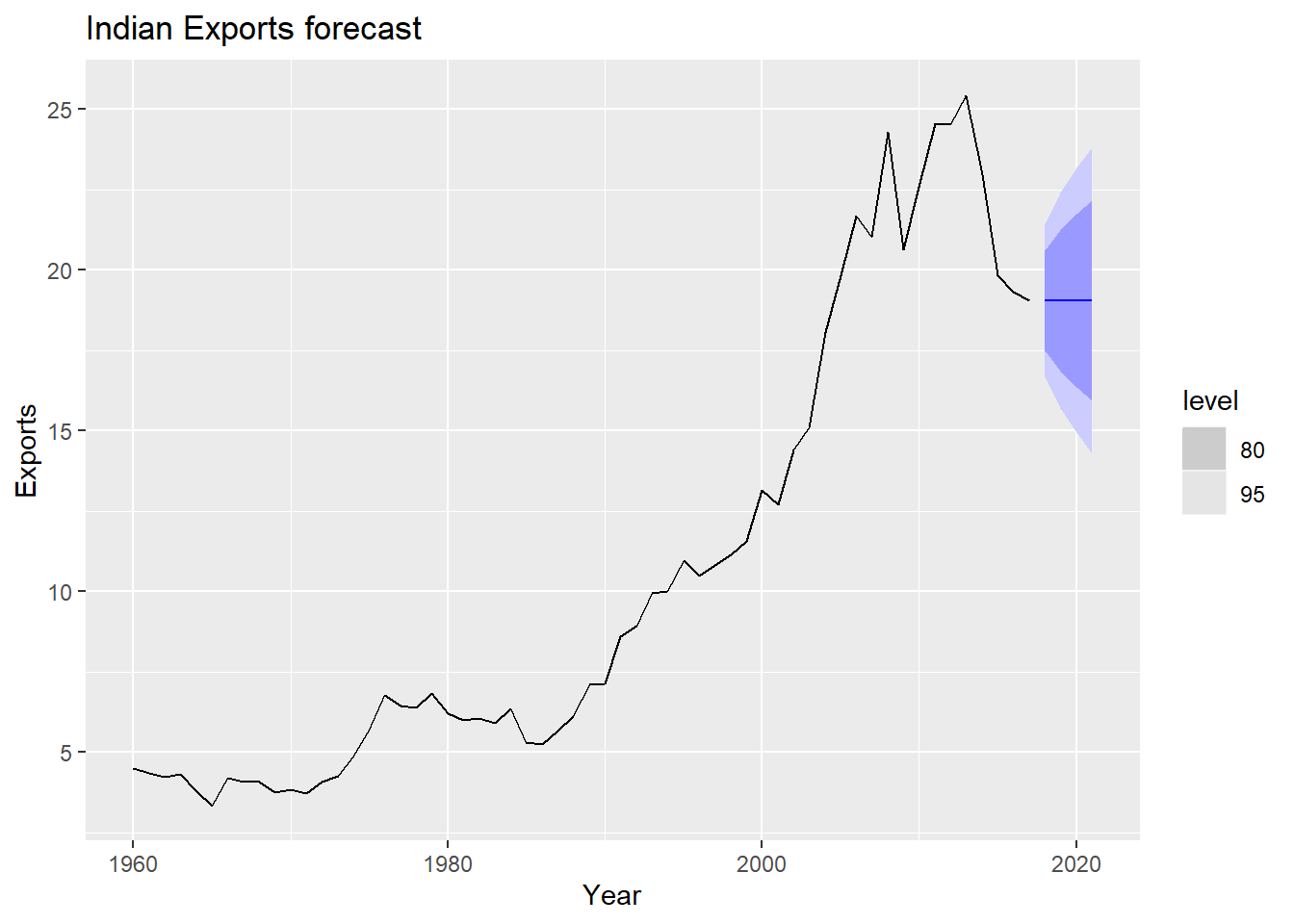###c) Compute the RMSE values for the training data.

##  1.191728

###d) Compare the results to those from an ETS(A,A,N) model. (Remember that the trended model is using one more parameter than the simpler model.) Discuss the merits of the two forecasting methods for this data set.

## Series: Exports
## Model: ETS(A,A,N)
##   Smoothing parameters:
##     alpha = 0.8289068
##     beta  = 0.2270934
##
##   Initial states:
##      l       b
##  4.598138 -0.1008885
##
##   sigma^2:  1.4854
##
##      AIC     AICc      BIC
## 264.3120 265.4659 274.6143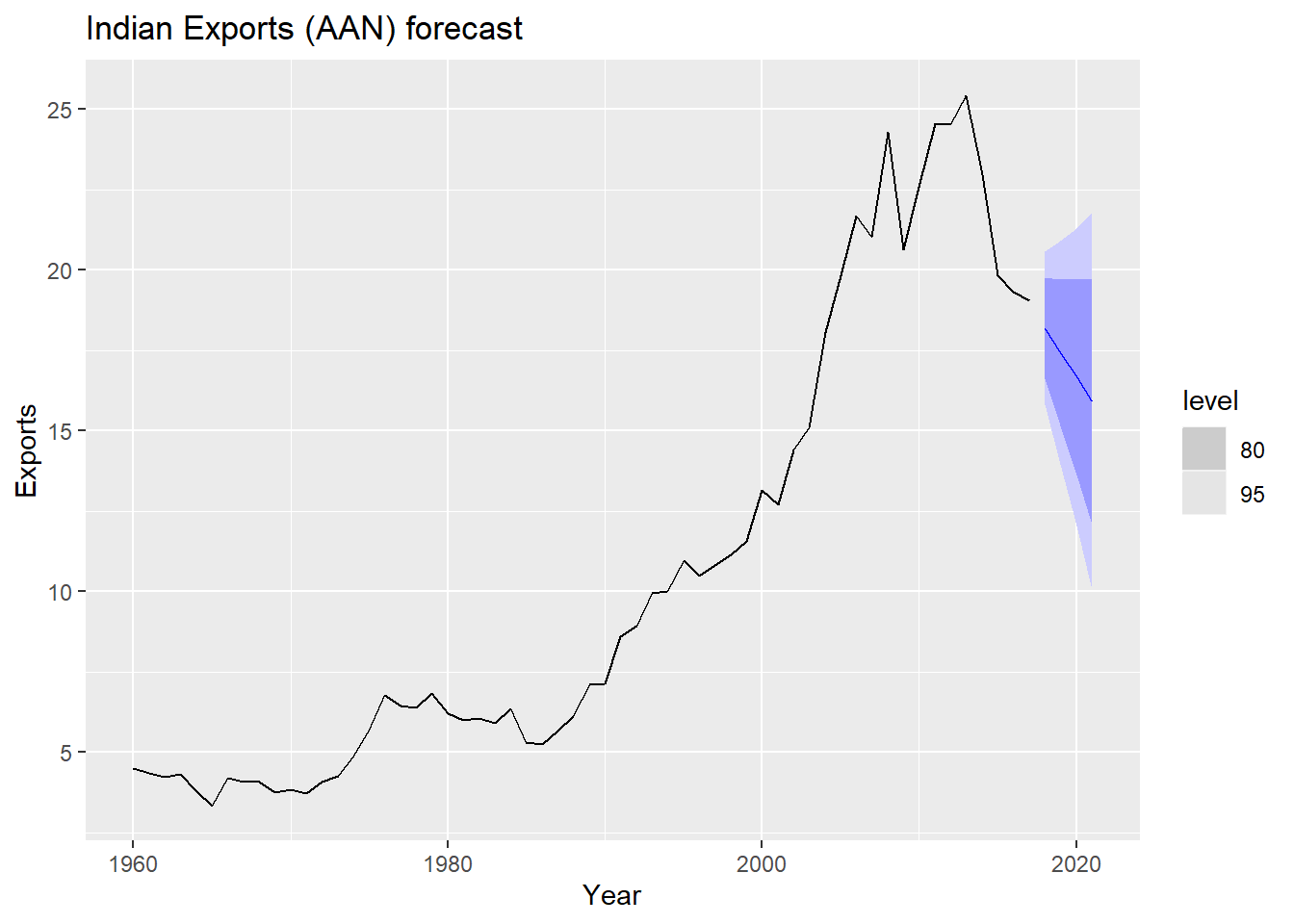###e) Compare the forecasts from both methods. Which do you think is best?

##  1.176006

The accuracy of the second model (AAN) with a trend component is much better than the previous SES model. The alpha parameter of the AAN model is lower than the alpha parameter of the ANN model, indicating that it would react slower to recent data points compared to the ANN model. Given that the AAN model incorporates a trend component and the exports time series was declining during the most recent years, the forecast of AAN is lower than the ANN model.

###f) Calculate a 95% prediction interval for the first forecast for each model, using the RMSE values and assuming normal errors. Compare your intervals with those produced using R.

## # A tsibble: 1 x 4 [1Y]
##    Year .mean `95%_lower` `95%_upper`
##   <dbl> <dbl>       <dbl>       <dbl>
## 1  2018  19.0        16.7        21.4
## # A tsibble: 1 x 4 [1Y]
##    Year .mean `95%_lower` `95%_upper`
##   <dbl> <dbl>       <dbl>       <dbl>
## 1  2018  18.2        15.8        20.6
##  1.191728
##  21.38118
##  16.7096
##  1.176006
##  20.49302
##  15.88308

As expected, the point forecast for the AAN model is lower than that of the ANN model due to the declining trend component in recent years, and the slightly lower RMSE for the AAN model results in a narrower band for the 95% confidence interval as compared to the AAN model.

##8.6. Forecast the Chinese GDP from the global_economy data set using an ETS model. Experiment with the various options in the ETS() function to see how much the forecasts change with damped trend, or with a Box-Cox transformation. Try to develop an intuition of what each is doing to the forecasts. [Hint: use a relatively large value of h when forecasting, so you can clearly see the differences between the various options when plotting the forecasts.]

## # A tsibble: 58 x 2 [1Y]
##      GDP  Year
##    <dbl> <dbl>
##  1  59.7  1960
##  2  50.1  1961
##  3  47.2  1962
##  4  50.7  1963
##  5  59.7  1964
##  6  70.4  1965
##  7  76.7  1966
##  8  72.9  1967
##  9  70.8  1968
## 10  79.7  1969
## # ... with 48 more rows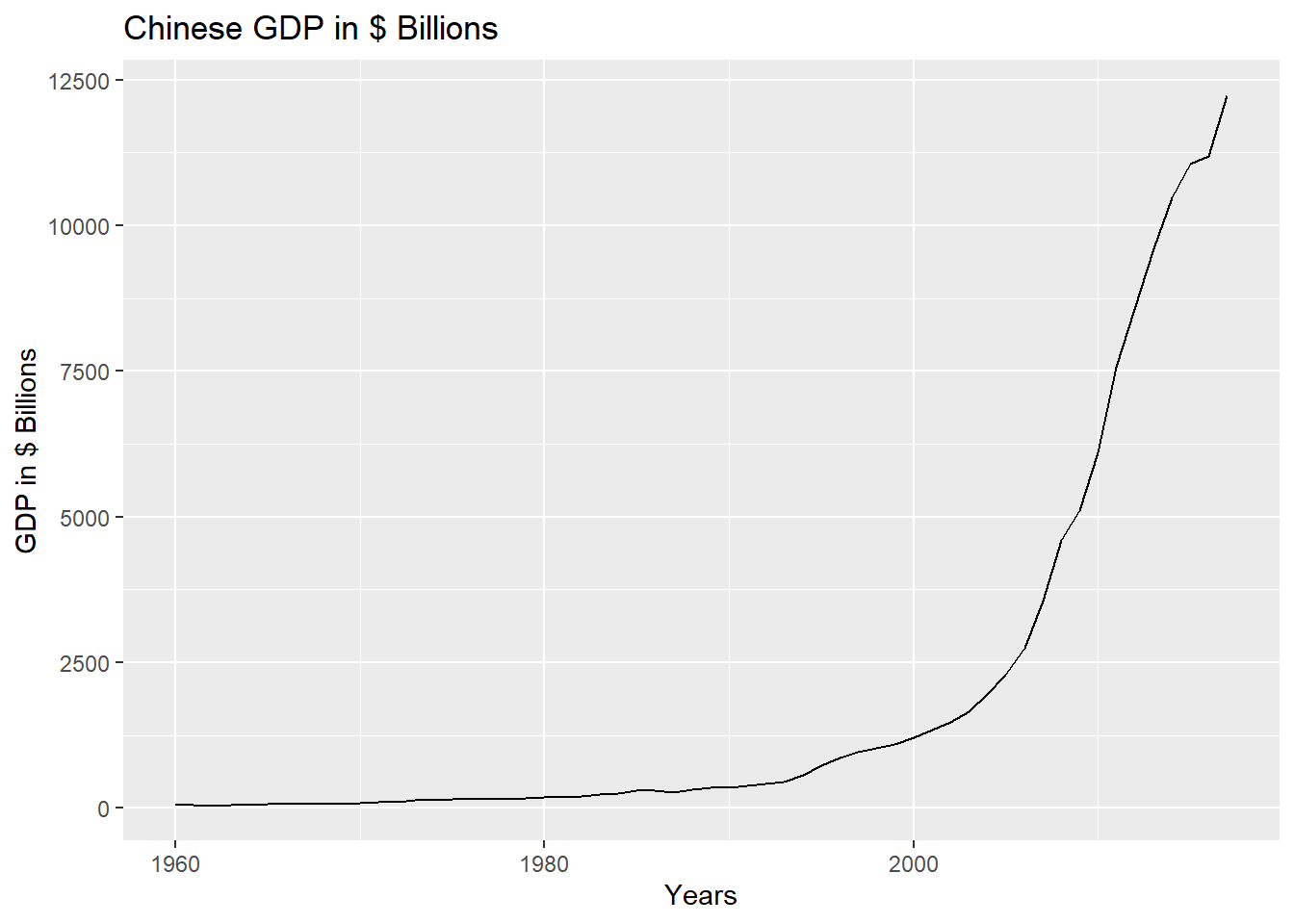From the plot above, we can see that the GDP grew very slowly from 1960 to around 1990, and increased faster from 1990 to 2000, after which it increased very sharply till the end of the observation period in 2017. So there is a clear trend in the time series, but there is no seasonality or cyclicality being shown.

Let’s decompose the time-series using the STL method.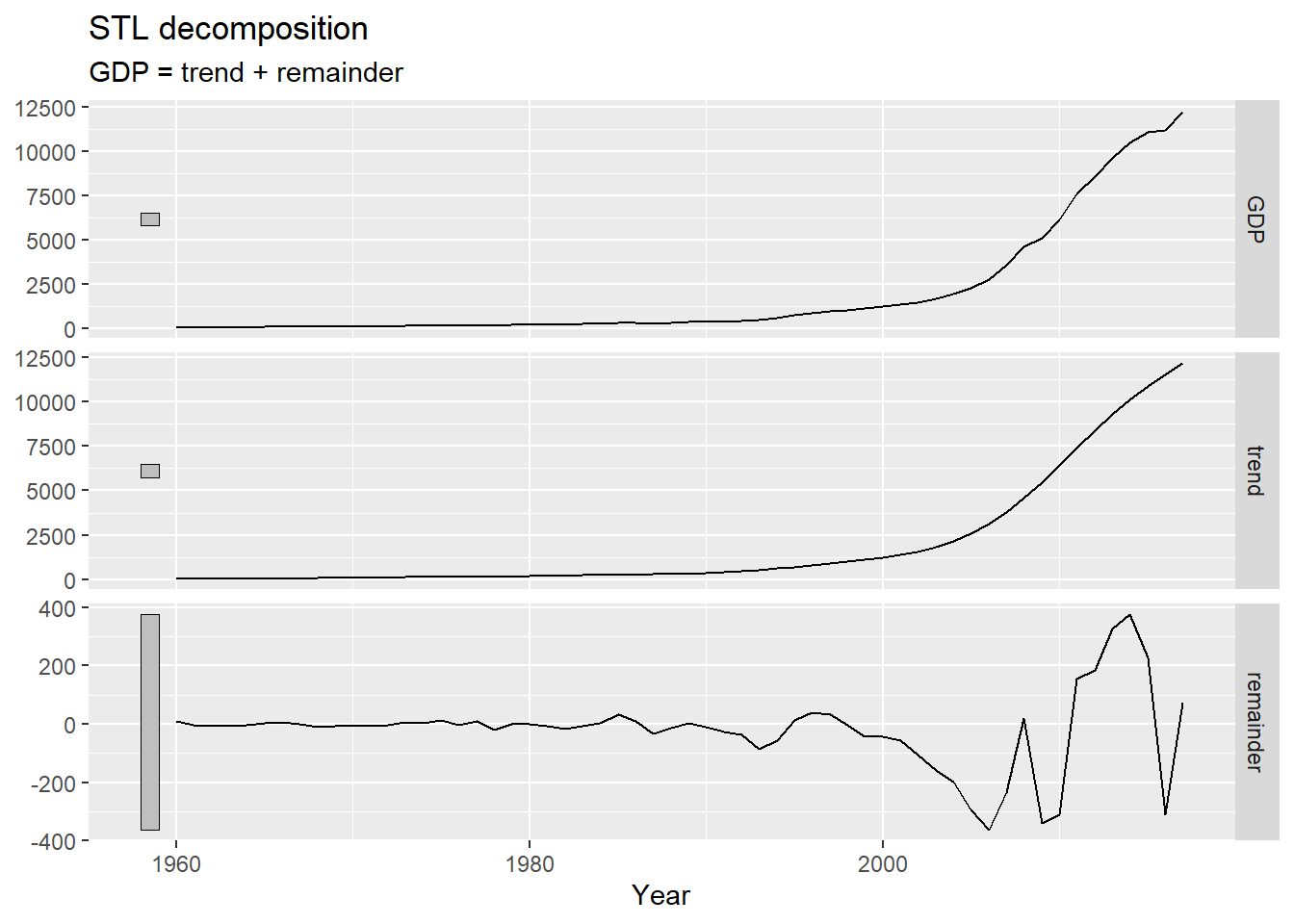The STL decomp shows the trend component that seems to the follow the underlying time series very well, but the remainder has increased in variance quite a lot after the year 2000. We could try to apply a Box Cox transformation to the time series to see if that stabilizes the variance of the remainder.

##  0.7730217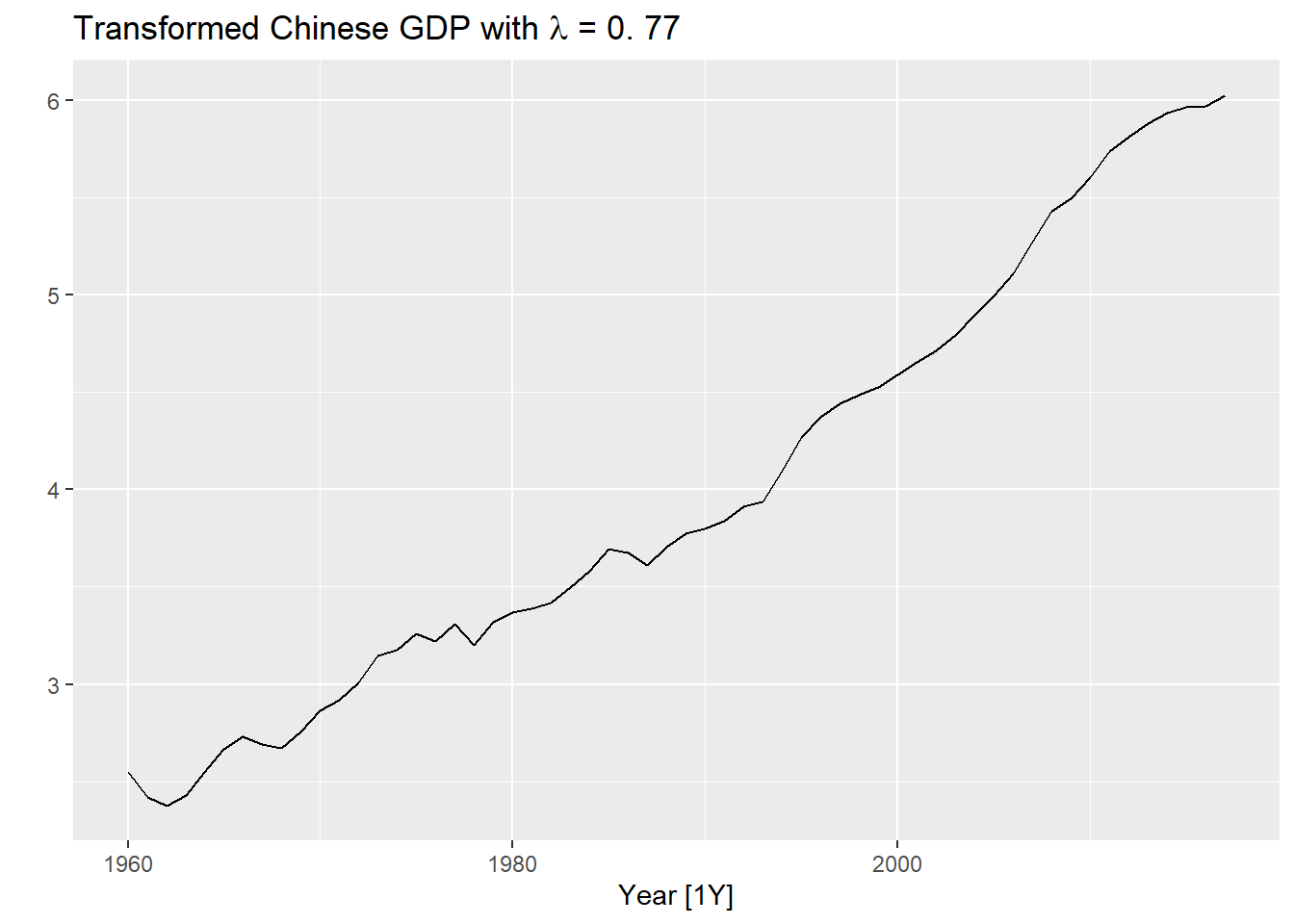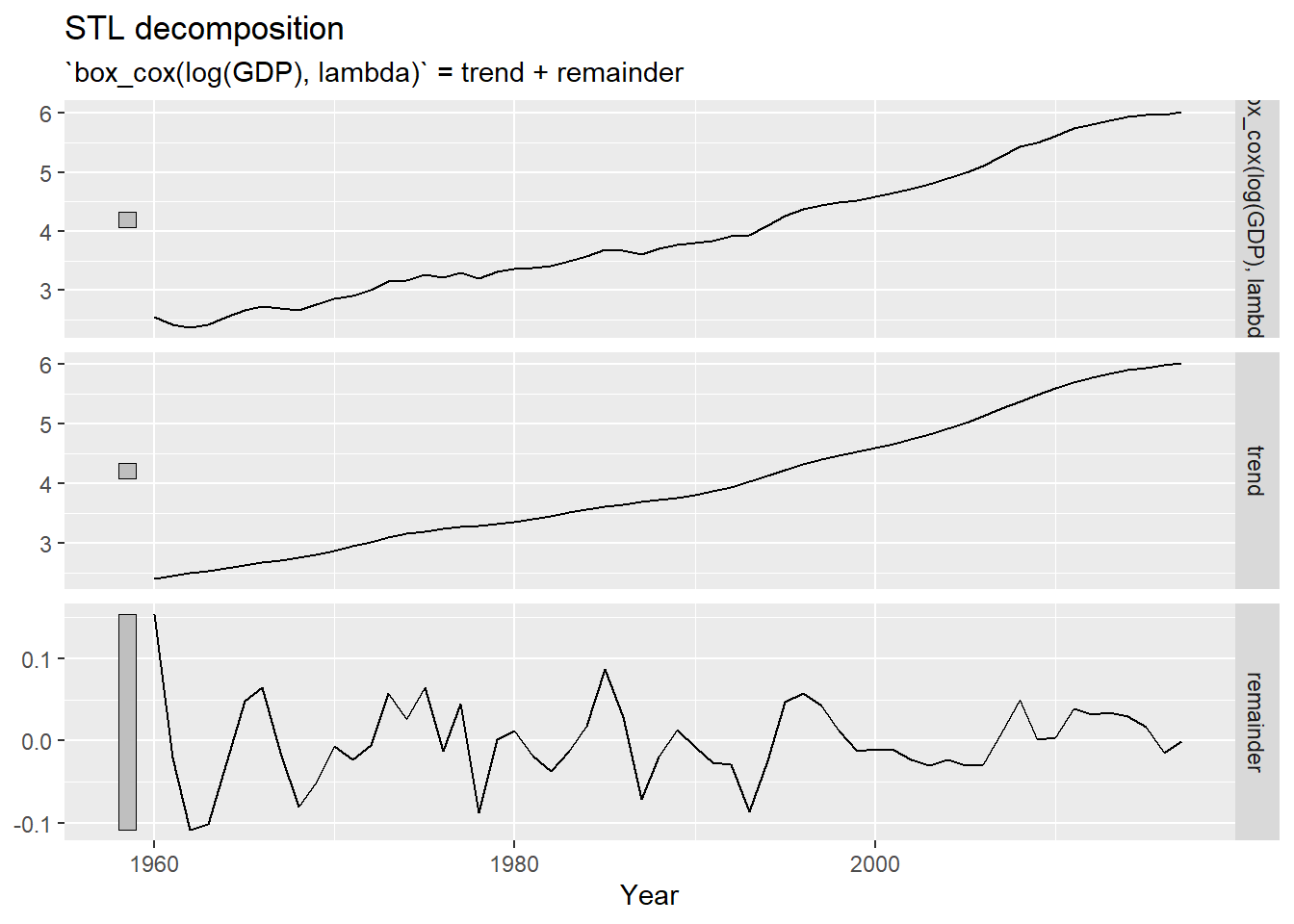Applying the Box Cox transformation to log GDP seems to have stablized the variance in the recent years.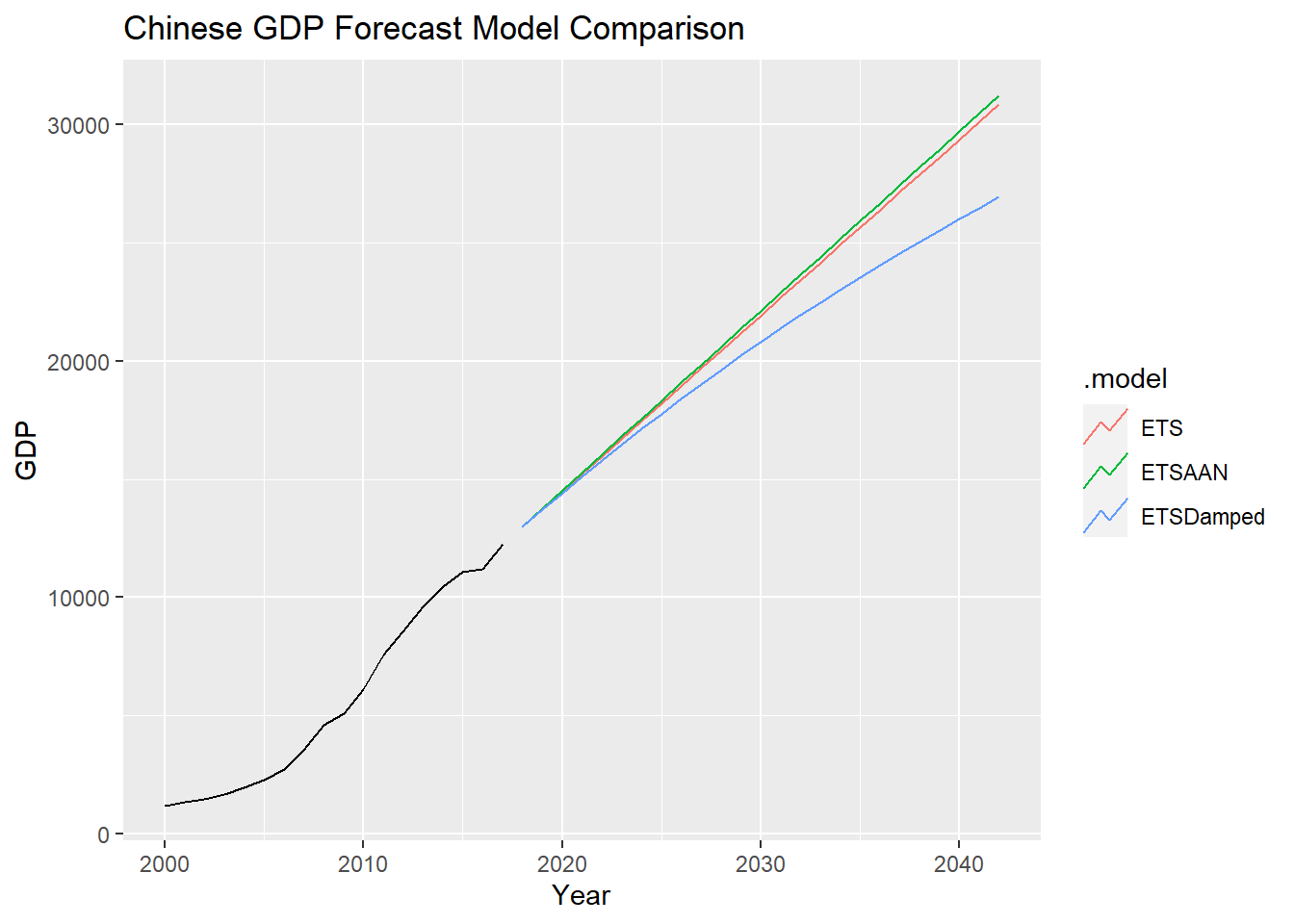## # A tibble: 5 x 9
##   .model         sigma2 log_lik   AIC  AICc   BIC         MSE       AMSE     MAE
##   <chr>           <dbl>   <dbl> <dbl> <dbl> <dbl>       <dbl>      <dbl>   <dbl>
## 1 ETS           0.00979  -341.  692.  694.  703.  38039.         1.50e+5  0.0754
## 2 ETSLog        0.00881    21.5 -33.1 -31.9 -22.8     0.00820    2.30e-2  0.0722
## 3 ETSBoxCox     0.00407    43.9 -77.8 -76.7 -67.5     0.00379    1.00e-2  0.0479
## 4 ETSAAN    38771.       -422.  854.  855.  864.  36097.         1.31e+5 96.0
## 5 ETSDamped 39589.       -422.  856.  858.  869.  36176.         1.29e+5 94.8
## # A tibble: 5 x 10
##   .model    .type       ME  RMSE   MAE   MPE  MAPE  MASE RMSSE     ACF1
##   <chr>     <chr>    <dbl> <dbl> <dbl> <dbl> <dbl> <dbl> <dbl>    <dbl>
## 1 ETS       Training  34.6  195.  95.1 2.20   7.27 0.439 0.465  0.194
## 2 ETSLog    Training -35.2  287. 125.  0.733  7.21 0.578 0.684  0.654
## 3 ETSBoxCox Training -15.0  276. 120.  0.718  7.12 0.552 0.657  0.648
## 4 ETSAAN    Training  23.6  190.  96.0 1.36   7.72 0.443 0.453  0.00882
## 5 ETSDamped Training  29.4  190.  94.8 1.86   7.56 0.437 0.454 -0.00684

The RMSE of the AAN model is the lowest.

## Series: GDP
##   Smoothing parameters:
##     alpha = 0.9998977
##     beta  = 0.5693401
##     phi   = 0.9799975
##
##   Initial states:
##     l      b
##  54.5142 -1.056062
##
##   sigma^2:  39589.13
##
##      AIC     AICc      BIC
## 856.2829 857.9300 868.6456

The optimal alpha value (smoothing parameter for the level) for the ETS-Damped model is almost 1, indicating that the model fitted would like to place a very high weight on the most recent observation. The beta parameter (estimate of the trend) is 0.56 which seems in line with the sharp increase in GDP seen in the past 17 years or so.

In general, the ETS-Damped trend model seems to make the most intuitive sense since it tones down the red-hot growth in GDP seen in the past 17 years. A country’s GDP depends on many factors and there is a cross-dependency in the case of China on other countries due to its export-led growth, which is bound to face elements of cyclicality over a longer observation period.

##8.7 Find an ETS model for the Gas data from aus_production and forecast the next few years. Why is multiplicative seasonality necessary here? Experiment with making the trend damped. Does it improve the forecasts?

## # A tsibble: 218 x 2 [1Q]
##    Quarter   Gas
##      <qtr> <dbl>
##  1 1956 Q1     5
##  2 1956 Q2     6
##  3 1956 Q3     7
##  4 1956 Q4     6
##  5 1957 Q1     5
##  6 1957 Q2     7
##  7 1957 Q3     7
##  8 1957 Q4     6
##  9 1958 Q1     5
## 10 1958 Q2     7
## # ... with 208 more rows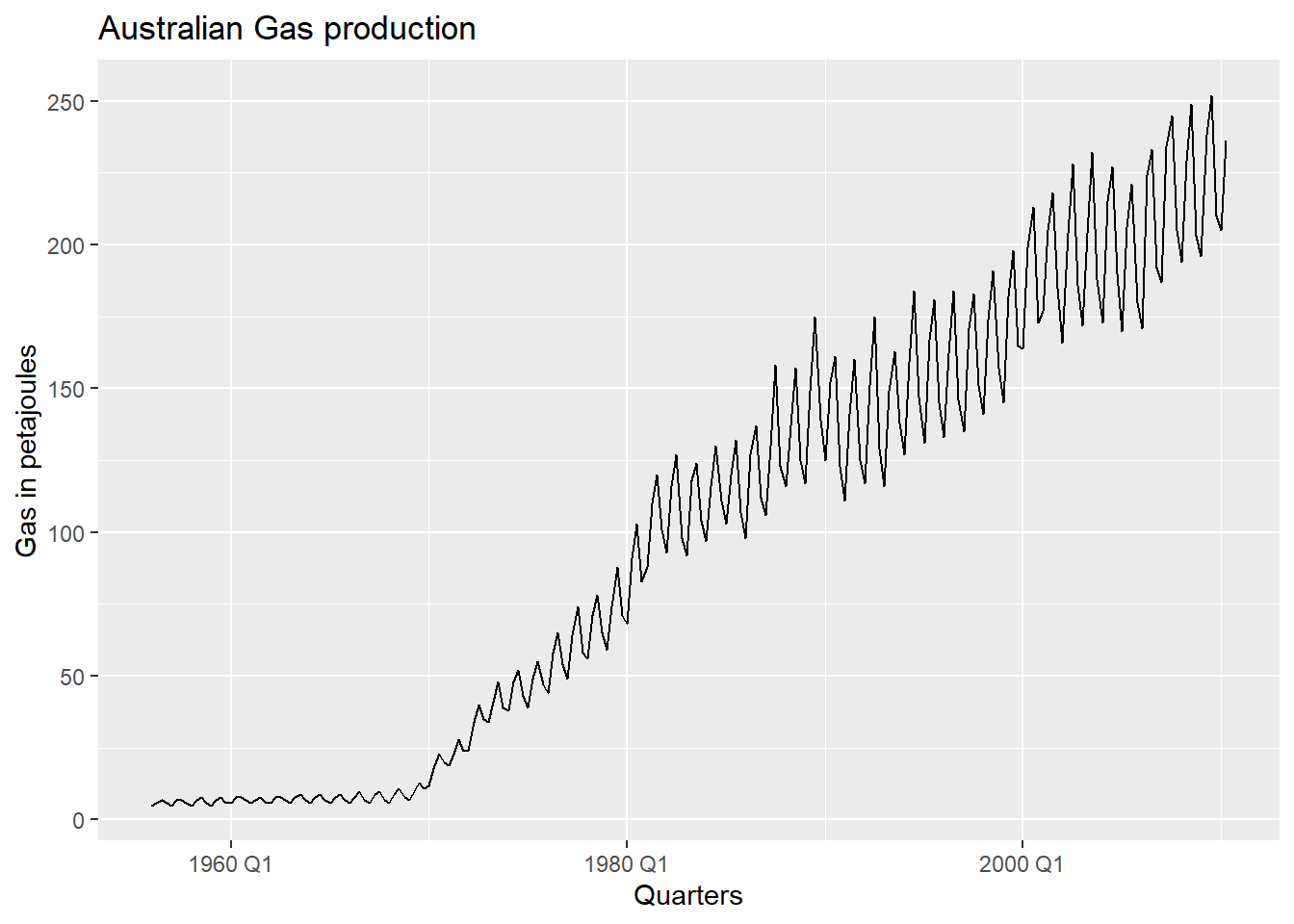The data shows an upward trend and strong seasonality. The variance also seems to have increased over the years. Let’s decompose the time series using the STL method.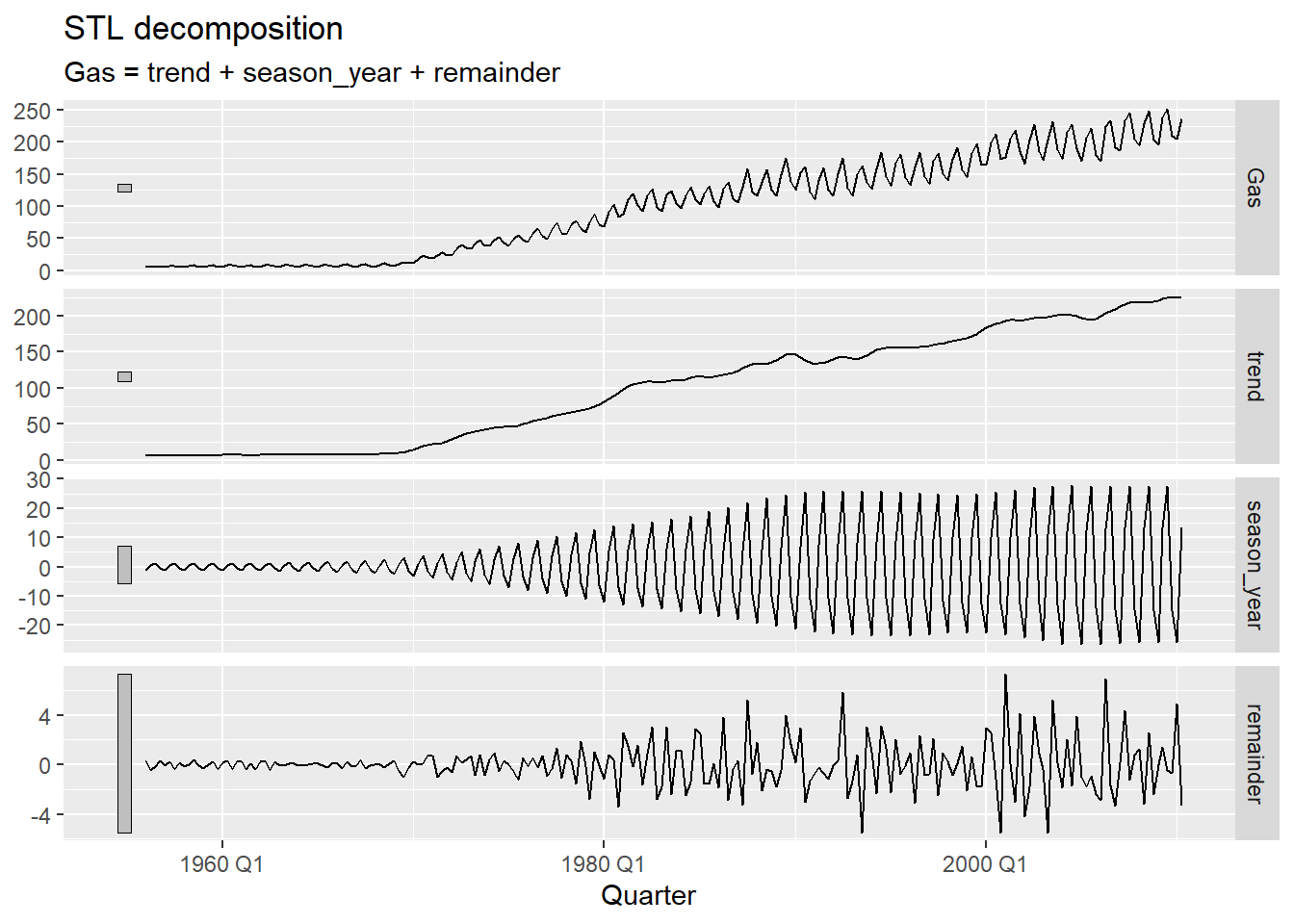As expected, the decomposition shows an upward trend, strong seasonality that seems to be time-dependent and a remainder that is increasing over time. Applying a model with multiplicative seasonality makes sense here since the seasonality component seems to be dependent on the overall level of the time series. we try fitting different models below including a damped trend model.

## # A tibble: 3 x 9
##   .model    sigma2 log_lik   AIC  AICc   BIC   MSE  AMSE   MAE
##   <chr>      <dbl>   <dbl> <dbl> <dbl> <dbl> <dbl> <dbl> <dbl>
## 1 ETSAAA      23.6   -927. 1872. 1873. 1903.  22.7  29.7  3.35
## 2 ETSAAM      18.2   -899. 1816. 1817. 1847.  17.6  25.1  2.84
## 3 ETSDamped   18.5   -901. 1821. 1822. 1855.  17.8  25.9  2.81
## # A tibble: 3 x 10
##   .model    .type         ME  RMSE   MAE    MPE  MAPE  MASE RMSSE   ACF1
##   <chr>     <chr>      <dbl> <dbl> <dbl>  <dbl> <dbl> <dbl> <dbl>  <dbl>
## 1 ETSAAA    Training 0.00525  4.76  3.35 -4.69  10.9  0.600 0.628 0.0772
## 2 ETSAAM    Training 0.218    4.19  2.84 -0.920  5.03 0.510 0.553 0.0405
## 3 ETSDamped Training 0.548    4.22  2.81  1.32   4.11 0.505 0.556 0.0265

The model parameters for the ETS-AAM model seem to provide the best fit. Let’s examine the forecasts.